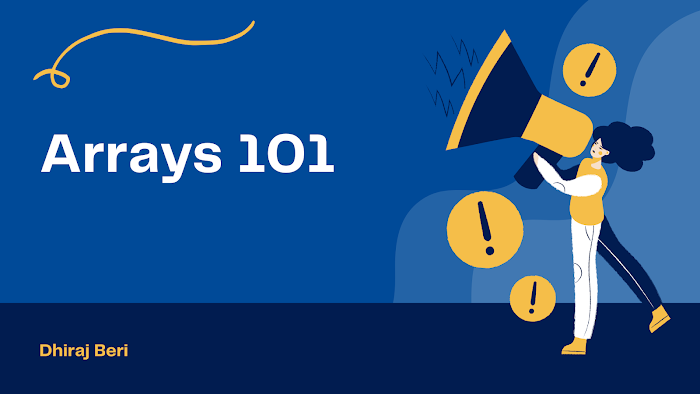# Finding Max and Min in a Single Scan

This is another great array question. Maximum and minimum of an array in a single scan.

For ex arr = 1,2,5,3 here minimum is 1 and maximum is 5.

`#include <stdio.h>int main(){    int a[] = {5, 8, 3, 9, 6, 2, 10, 7, -1, 4};    int min, max, i;    min = a;    max = a;    for (i = 1; i < 10; i++)    {        if (a[i] < min)            min = a[i];        else if (a[i] > max)            max = a[i];    }    printf("%d %d\n", min, max);    return 0;}`

First of all, we take array a[] and initialize it. Then take three variables min and max and i for iterating the loop.

Initialize min and max with the first element of array.

Now take a loop from iterate over n elements.

Check if the element is smaller than the min then assign it to the min variable. And if a variable is larger than max then assign it to a max variable.

That's how we get out min and max in a single scan.

The time complexity of this approach is O(n). Have doubts? Let's discuss this in the comment section.